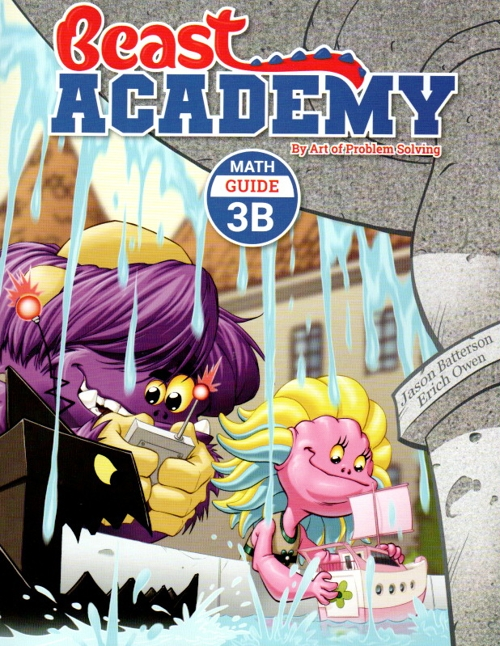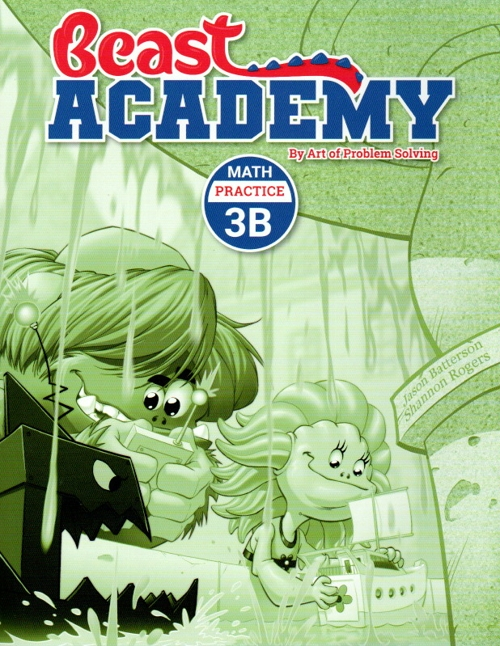Art of Problem Solving Beast Academy 3B Guide and Practice \$30 : Multiplication: The times table, the commutative and associative properties, multiplying numbers that end in one or more zeroes. Perfect Squares: Squaring a number that ends in 5, finding the next perfect square, multiplying nearby numbers using perfect squares. The Distributive Property: Order of operations, area models of the distributive property, multiplying multi-digit numbers.